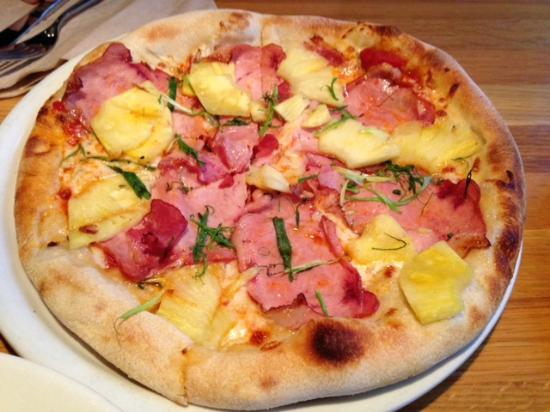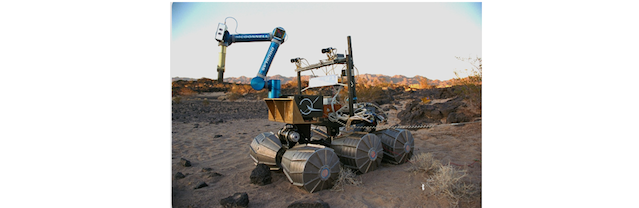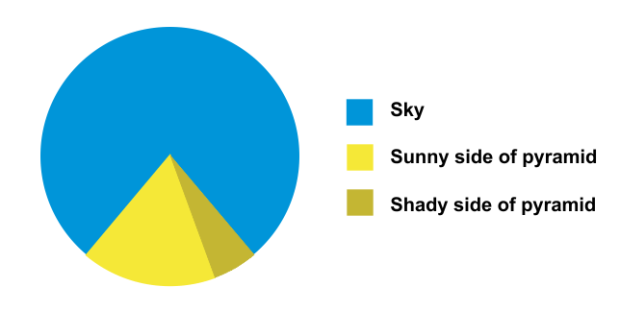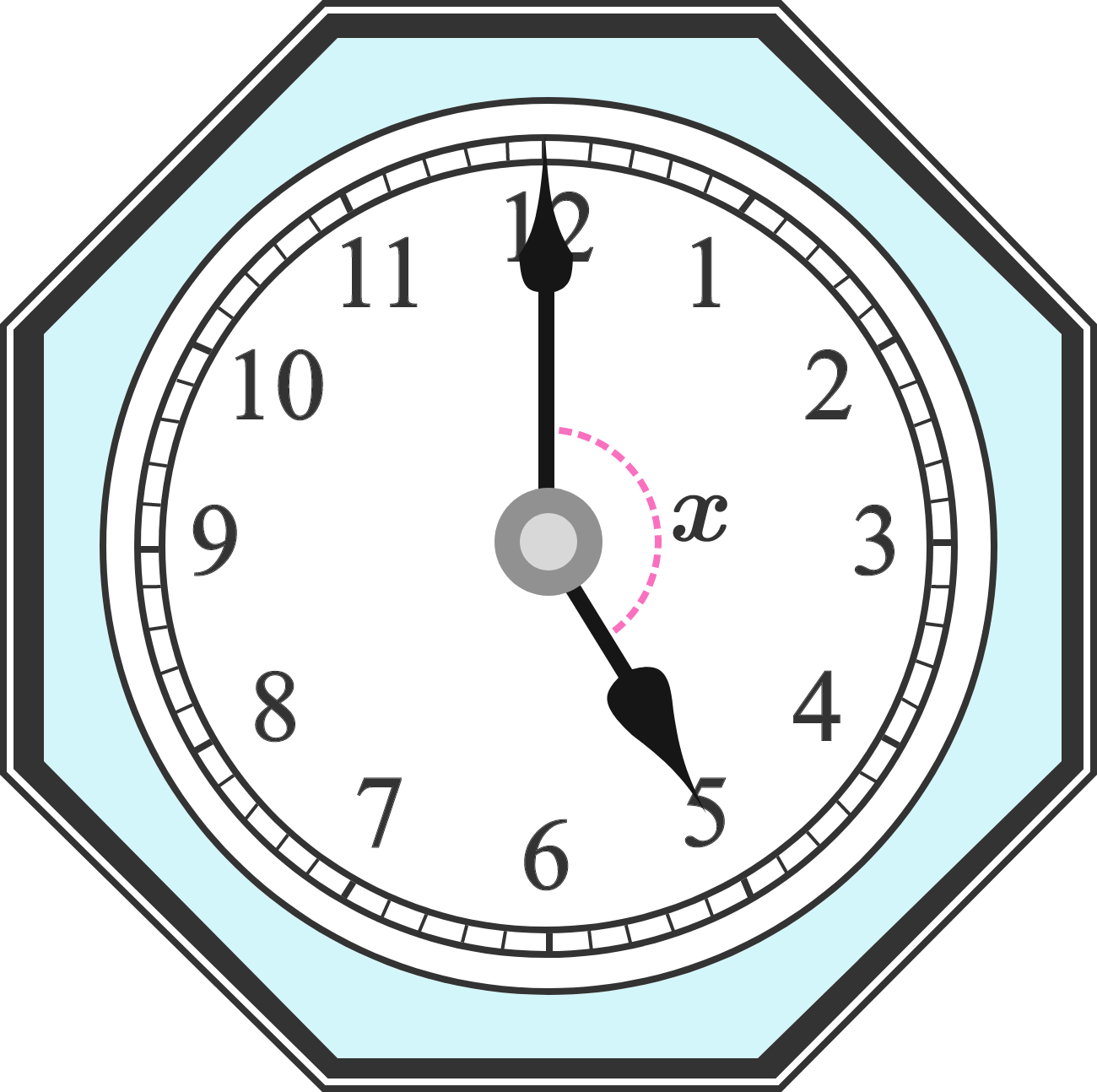Basic Mathematics

# Angles and Lines: Level 1 ChallengesYou order a pizza from a parlor with some friends. The chef has cut the round pie into four slices with two straight cuts that go through the center of the pizza. One of the slices is three times as large as the piece just next to it! What's the angle of the tip of the largest pizza slice in the box?True or False:

$\quad$ Two lines with positive slopes can be perpendicular.NASA is testing their space robot on a flat plane. They gave it instructions to:
- Move forward 3 miles
- Turn 90 degrees left
- Move forward 4 miles
- Turn 90 degrees left
- Move forward 3 miles

How far away will the robot be from its starting point?Consider the pie chart shown above, where the ratio of angles formed by the 3 sectors are $3 : 14 : 1$. In degrees, what is the measure of angle formed for the sector that represents the shady side of the pyramid?

###### Image Credit: Flowing Data.The clock shown has hands positioned at 5 o'clock. What is the degree measure of angle $x$?

×